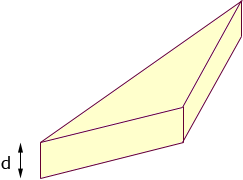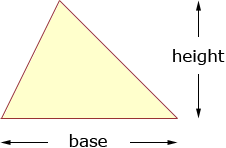Hello I have forgotten the exact formula for this. How do I find the volume of a triangular box? Can you help please? Thanks Irene Hi Irene, Is this what you mean by a triangular box?If so then the volume is the box is the area of the triangle times the depth, d. The area of the triangle you can find in two different ways. One is to choose a side of the triangle as the base and then the area is triangle area = 1/2baseheightAn alternate way to calculate the area of a triangle is to use Heron's Formula. Penny